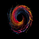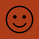10346 views
10346
This very simple indicator will give you a blue background where the CCI crossed from below -100 to above -100, and a red background where it crossed from above 100 to below 100.
```study("CCI Crossover Alert", overlay=true)
length=input(20, "Length", integer)

current = cci(close, length)
previous = cci(close, length)

bgcolor(current < 100 and previous > 100 ? red : na)
bgcolor(current > -100 and previous < -100 ? blue : na)

//plot(100, style=area, transp=95, color=blue)
//plot(-100, style=area, transp=95, color=blue)
//plot(cci(close, length))```Is there a scanner or something were I can get alerted when CCI (62) crosses 0 in a certain timeframe? Or could it be built into a script ?im trying to add alert to this CCI template i got form the website. i am getting errors. any help. i watn a alert every time it crosses the CCI. below or above the 100 or - 100

study("CCI Crossover Alert_V4", overlay=true)
length=input(20, "Length", integer)

current = cci(close, length)
previous = cci(close, length)

bgcolor(current < 100 and previous > 100 ? red : na)
bgcolor(current > -100 and previous < -100 ? blue : na)

alert1= bgcolor(current < 100 and previous > 100 ? 1 : 0
alert2= bgcolor(current > -100 and previous < -100 ? 1 : 0

//plot(100, style=area, transp=95, color=blue)
//plot(-100, style=area, transp=95, color=blue)
//plot(cci(close, length))hey thank you for posting this indicator, really helpful for me to learn coding and this is very simple yet useful! :) cheers!Thank you!

One question: It seems to not fit with the same data given by the TradingView build-in CCI indicator - when crossing from below -100 to above -100. The built-in commodity channel indicator shows those crossings very noticeable earlier than your version of the CCI. Please compare them yourself.ChartArt
Thanks for your comment. I don't quite understand what you mean though. As you can see, all I do is check if the built-in CCI is now above -100 and last period was below -100 (meaning it won't get triggered if it's exactly at -100).

Perhaps the problem is the painted background seems to come late because until the current candle isn't closed you can't be sure it has indeed crossed the threshold? I hope I'm explaining clearly. If I'm misunderstanding the problem please give me more details.Johnman
Yes, maybe the candle close is the problem.

Here is an example. I used your chart and switched to 5-minute (where I trade):

Your CCI indicator signals are always lagging behind the actual crossings, although you are using the build-in version.ChartArt
Yes, I think the problem is the signal is not given until the second candle (the one where the crossing happens) is closed. This means the signal is given on the opening of the third candle (first candle closes below, second candle closes above, third candle opens and signal appears). That is intentional because the second candle could reverse and close below, thus the signal not being confirmed.

Maybe you can alleviate this by modifying the code so it uses opening prices instead of closing prices, but that will also mean more false signals.

I'm a fundamental trader anyway, I only did this so I could learn a bit about programming indicators in TradingView so I really can't guarantee this usefulness of this. :PJohnman
I tried it before. When I changed:

current = cci(close, length)
previous = cci(close, length)

to

current = cci(open, length)
previous = cci(open, length)

The colored signal comes even one bar later than with the "close" setting. Don't ask me why :)